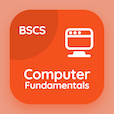Colleges Online Courses

College Math MCQs

College Math MCQ PDF - Topics

# Adjoint and inverse of square Matrix MCQ Quiz Online

Practice Adjoint and inverse of square Matrix Multiple Choice Questions (MCQ), Adjoint and inverse of square Matrix quiz answers PDF to learn college math online course for college math classes. Matrices and Determinants Multiple Choice Questions and Answers (MCQs), Adjoint and inverse of square Matrix quiz questions for online college for teaching degree. "Adjoint and inverse of square Matrix MCQ" PDF Book: symmetric matrix, homogeneous linear equations, multiplication of a matrix, column matrix test prep for best online GRE prep class.

"The additive inverse of a matrix A is" MCQ PDF: adjoint and inverse of square matrix with choices a, |a|, a², and adj a⁄|a| for online college for teaching degree. Learn adjoint and inverse of square matrix quiz questions for merit scholarship test and certificate programs for best accredited online colleges.

## MCQs on Adjoint and inverse of square Matrix Quiz

MCQ: The additive inverse of a matrix A is

A
|A|

MCQ: If AB exists, then ( AB )-1is

A-1 B-1
B-1 A-1
AB
None of Above

### More Topics from College Math Course